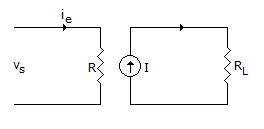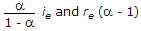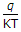# Electronics and Communication Engineering - Analog Electronics

16.

Which one of the following power amplifier has the maximum efficiency?

 A. Class A B. Class B C. Class AB D. Class C

Explanation:

No answer description available for this question. Let us discuss.

17.

Figure shows small signal model of CB amplifier circuit. Then I and R are respectively equal toA. a ie and re B.C. a ie and re (a - 1) D. (a - 1) ie and re

Explanation:

No answer description available for this question. Let us discuss.

18.

The common emitter short circuit current gain β of a transistor

 A. is a monotonically increasing function of the collector current Ic B. is a monotonically decreasing function of Ic C. increasing with Ic, for low Ic, reaches a maximum and then decreases with further increase in Ic D. is not a function of Ic

Explanation:

No answer description available for this question. Let us discuss.

19.

A complementary symmetry amplifier has

 A. 1 P-N-P and 1 N-P-N transistor B. 2 P-N-P transistor C. 2 N-P-N transistor D. 2 P-channel FETs

Explanation:

No answer description available for this question. Let us discuss.

20.

The units ofare

 A. V B. V-1 C. J D. J/K